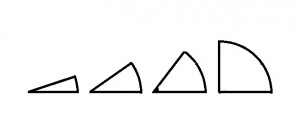# Measuring Angles

If you have been following my recent posts, you may now be waiting for an impassioned defense of “completing the square”.  It’s coming soon, with pictures (of course).  But talking about the aspect ratio of a rectangle got me thinking about other ratios that show up in geometry. In particular, I want to look at what we might call the aspect ratio of an angle.  This will lead us to a natural way of describing the size of an angle, one that is less arbitrary than the degree system.  So I have interrupted the regularly scheduled digression for a different digression.

Measuring Angles the Familiar Way

When two rays intersect, we say that they form an angle.  For example, the two rays could be cut along different radii of a pizza, intersecting at the center of the pie.  Depending on how you make your cuts, you will get different sizes for your central angle.  If you are hungrier, you cut a larger central angle.Since angles come in different sizes, we need to have a system for measuring them.  And of course, we have a system that you have known forever.  We call a full circle 360 degrees.  We call a u-turn a “180”, short for 180 degrees.  We call the central angle of a quarter of a pizza a 90-degree angle.  That is also the measure of the angle you find in the corner of every rectangle.  It’s such a familiar system that you may not even realize that it is completely arbitrary.  Who chose 360 as the magic number for a full circle?  (I believe it goes back to the ancient Sumerians, but I haven’t even checked Wikipedia.)  My point is that we didn’t have to do it this way (and we don’t always).

Another way we could measure angles

I’m just saying that this would work too:

Let’s describe the size of an angle by comparing it to a right angle, defining the measure of each angle as a percent of the measure of a right angle.  So for example, the angle made by the diagonal of a square is half the size of a right angle.  You are used to calling that a 45 degree angle, but in our new system, that would be an angle measure of 50 — the diagonal makes 50% of a right angle.Here are some fun facts about our new system:

1. A right angle has measure of 100. (It is 100% of a right angle!)

2. A full rotation has measure 400. (Cut a pizza into 4 quarters.  The four right angles add up to a complete circle.

3. The measures of the angles of a triangle still add up to the same as the measure of a straight line.  They each have a total measure of 200 in this system.

4. Equilateral triangles have 3 angles with the same measure: 200/3 = 66.666…

This seems arbitrary and unfamiliar – compared to our current system which is arbitrary but at least familiar.  But here’s one other fun fact about our new system:

5.  It isn’t new at all!  It’s an existing system.  When you express an angle as a percent of a right angle, you are measuring the angle in “gradians” or “grads”.  This a real thing. It may even be one of the modes on your calculator (but no one ever uses it).

I’ve read that gradians were used in navigation but I have never seen it done.  To me, it feels like a quirk of history that we chose one arbitrary system over another.  There is, however, another way to measure the size of an angle that is not arbitrary at all.  It is a natural way to describe different angles using ratios in a manner much like the way they are used to describe rectangles.  This system has a real name: it’s called “radian measure” (and your calculator has a mode for this as well).  But we can also think of it as the “Angular Aspect Ratio”.   I’ll describe how it works in my next post.